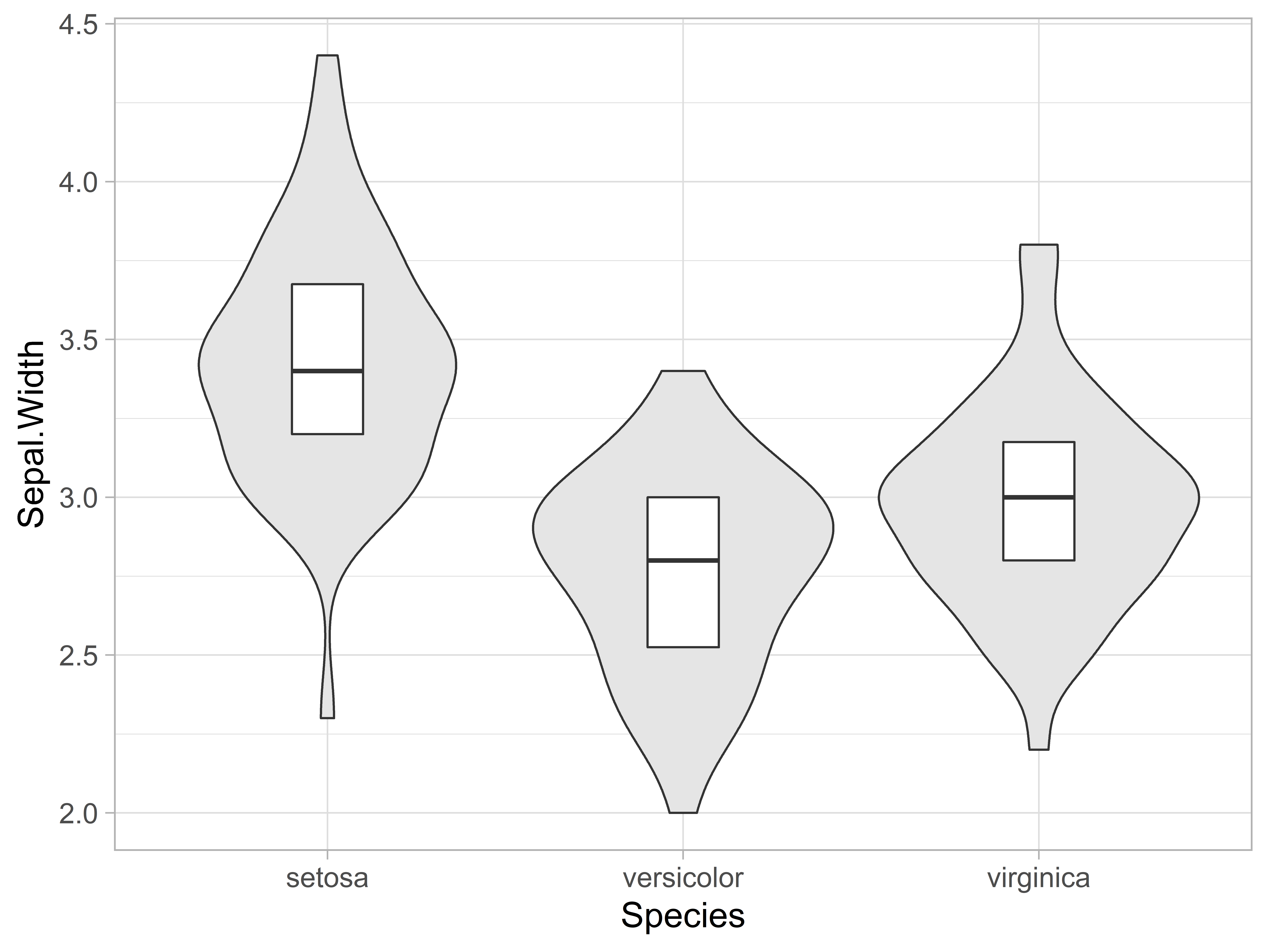# Raincloud Plots with ggplot2

Cédric Scherer https://cedricscherer.com
2021-03-23

# Packages

``````library(ggplot2)
library(ggforce)
library(ggdist)
library(gghalves)

theme_set(theme_light(base_size = 16))
``````

# Plots

## Violin-boxplot-combination

``````g1 <-
ggplot(iris, aes(Species, Sepal.Width)) +
geom_violin(fill = "grey90") +
geom_boxplot(width = .2, outlier.shape = NA, coef = 0)

g1
````````````g1 + geom_point(alpha = .7, position = position_jitter(seed = 1))